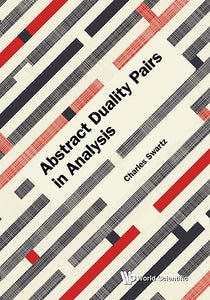# Abstract Duality Pairs In Analysis

Charles W Swartz

Format: Print Book

ISBN: 9789813232761

• SGD 187.25
Unit price per
Tax included.

The book presents a theory of abstract duality pairs which arises by replacing the scalar field by an Abelian topological group in the theory of dual pair of vector spaces. Examples of abstract duality pairs are vector valued series, spaces of vector valued measures, spaces of vector valued integrable functions, spaces of linear operators and vector valued sequence spaces. These examples give rise to numerous applications such as abstract versions of the Orlicz-Pettis Theorem on subseries convergent series, the Uniform Boundedness Principle, the Banach-Steinhaus Theorem, the Nikodym Convergence theorems and the Vitali-Hahn-Saks Theorem from measure theory and the Hahn-Schur Theorem from summability. There are no books on the current market which cover the material in this book. Readers will find interesting functional analysis and the many applications to various topics in real analysis.

Format: Hardcover
No of Pages: 304
Imprint: World Scientific
Publication date: 20171221